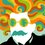# How to Solve It?

How can we prove that-

$n \times (n^{2} + 20)$ is divisible by 48.

[Given : n is a positive even number]Note by Vatsalya Tandon
6 years, 3 months ago

This discussion board is a place to discuss our Daily Challenges and the math and science related to those challenges. Explanations are more than just a solution — they should explain the steps and thinking strategies that you used to obtain the solution. Comments should further the discussion of math and science.

When posting on Brilliant:

• Use the emojis to react to an explanation, whether you're congratulating a job well done , or just really confused .
• Ask specific questions about the challenge or the steps in somebody's explanation. Well-posed questions can add a lot to the discussion, but posting "I don't understand!" doesn't help anyone.
• Try to contribute something new to the discussion, whether it is an extension, generalization or other idea related to the challenge.

MarkdownAppears as
*italics* or _italics_ italics
**bold** or __bold__ bold
- bulleted- list
• bulleted
• list
1. numbered2. list
1. numbered
2. list
Note: you must add a full line of space before and after lists for them to show up correctly
paragraph 1paragraph 2

paragraph 1

paragraph 2

[example link](https://brilliant.org)example link
> This is a quote
This is a quote
    # I indented these lines
# 4 spaces, and now they show
# up as a code block.

print "hello world"
# I indented these lines
# 4 spaces, and now they show
# up as a code block.

print "hello world"
MathAppears as
Remember to wrap math in $$ ... $$ or $ ... $ to ensure proper formatting.
2 \times 3 $2 \times 3$
2^{34} $2^{34}$
a_{i-1} $a_{i-1}$
\frac{2}{3} $\frac{2}{3}$
\sqrt{2} $\sqrt{2}$
\sum_{i=1}^3 $\sum_{i=1}^3$
\sin \theta $\sin \theta$
\boxed{123} $\boxed{123}$

Sort by:

Since n is even, Let n=2k for some k.

Then, The Expression becomes,

$2k({(2k)}^{2}+20)$ = $8k({k}^{2}+5)$

Thus, 8 is a factor. Now, we know that one of k or ${k}^{2}+5$ has to be a multiple of 2. Hence We get that 2 is also a factor.

let $n({n}^{2}+20)= {n}^{3}+20n$.

One can see that, ${n}^{3}=n (mod 3)$. So, ${n}^{3}+20n$ = n+20n =21n Which is congruent to 0 (mod 3)

Hence, We find that 3 is also a factor. So, The number is completely divisible by $8*2*3=48$.

Hence, Proved :D

This was my first Proof. If you spot any mistakes, Kindly inform it to me. I will clean it up.

- 6 years, 3 months ago

Cheers!!! xD

- 6 years, 3 months ago

LOL, Cheers! xD

- 6 years, 3 months ago

Good!

- 6 years, 3 months ago

Thanks! :D

- 6 years, 3 months ago

Let n be 2k :

Then, $2k(4k^{2} +20)$

$8k^{3}+40k$

$8k(k^{2}+5)$

$8k(k^{2}-1+6)$

$8k((k+1)(k-1)+6)$

$8(k-1)(k)(k+1)+8k \times 6$

$8(k-1)(k)(k+1)+48k$

Now, $48k$ is clearly divisible by 48 and,

$(k-1)(k)(k+1)$ are three consecutive numbers so they will be divisible by $3! = 6$ AND $(k-1)(k)(k+1)$ is multiplied by 8 so the product will be divisible by $8 \times 6$ which is $48$.

It is proved now that $8(k-1)(k)(k+1)$ and $48k$ are both divisible by 48 and when you add two numbers which are divisible by 48, obviously the sum will also be divisible by 48.

- 6 years, 3 months ago

Yeah even i did the same way

- 5 years, 2 months ago

Sorry Guys! Updated the Values! Sorry for the Inconvenience!

- 6 years, 3 months ago

Ok I Finally Get This-

Let us assume $n$ to be an even number $2k$. Now, the equation becomes- $2k(4k^{2}+20)$ and then $8k^{3}+40k$. Taking $8k$ to be common like this- $8k(k^{2} + 5)$, we can split "5" in the equation to form this- $8k(k^{2} + 6 - 1)$. And factorizing $k^{2} + 1$ gives us, $8k((k+1)(k-1) + 6)$. Here we would be using an identity that the product of $n$ consecutive numbers is divisible by $n!$. Thus in this case we have 3 consecutive numbers- $k, k+1, k-1$, which means that this part is divisible by $6$. So, this part- $8 * (k) * (k+1) * (k-1)$ is divisible by $48$ (as we have 8 multiplied to it and 6 divides it). Now the remaining part is- $8k*6$ which in itself is divisible by 48. Thus the sum of 2 numbers divisible by 48 will give a number divisible by 48.

Q.E.D (Hope if you Like it!). And thanks to @MehulArora for his initial solution. Cheers!

- 6 years, 3 months ago

My Pleasure @Vatsalya Tandon

- 6 years, 3 months ago

Taking $n=2x$ and simplifying a bit gives,$8(x^3+5x)$.Now,we just need to prove that $x^3+5x\equiv 0\pmod{6}$.For doing that we first write $x(x^2+5)\\ =x(x^2+6-1)\\ =x^3+6x-x\\ =x^3-x+6x\\ x(x^2-1)+6x\\ (x-1)(x)(x+1)+6x$.Now,we just need to prove that $(x-1)(x)(x+1)\equiv 0\pmod{6}$ which is obvious.Hence proved.@Vatsalya Tandon

- 6 years, 3 months ago